# GRE Subject Test: Biology : Understanding Hardy-Weinberg Equilibrium

## Example Questions

### Example Question #1 : Understanding Hardy Weinberg Equilibrium

In the Hardy-Weinberg equations, what quantities are represented by the variablesand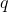?

Allele frequencies

Observed phenotype frequencies

Genotype frequencies

Expected phenotype frequencies

Allele frequencies

Explanation:

The variablesandare specifically referring to the allele frequencies of the dominant and the recessive allele in a population, respectively.

Expected genotype frequencies can be seen in the equation: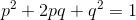In this equation,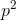represents the expected genotype frequency of homozygous dominant organisms,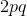represents the expected frequency of heterozygous organisms, and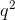represents the frequency of homozygous recessive organisms. These values are the expected frequencies in the population, based on the Hardy-Weinberg conditions and allele frequencies; they may not be the values actually observed. To get observed genotype and phenotype frequencies, more information about the size and makeup of the population would be needed.

### Example Question #1 : Understanding Hardy Weinberg Equilibrium

Which of the following variables would not be observed in a population at Hardy-Weinberg equilibrium?

The population has a very large number of organisms

Females mate with males of the same color to avoid a mixing of colors in the population

No immigrating organisms are allowed to enter the population

No new mutations are appearing in the population

Females mate with males of the same color to avoid a mixing of colors in the population

Explanation:

Hardy Weinberg equilibrium has requirements that must be met by a population in order to confirm that evolution is not taking place:

1. The population must be large in number.

2. There can be no new mutations entering the population.

3. Immigration and emigration cannot change the allelic frequencies of the population.

4. Mating must be random.

5. Natural selection cannot be taking place.

Since it was said that females are selectively choosing which males they mate with, Hardy Weinberg equilibrium is being violated.

### Example Question #1 : Evolution And Mutations

A population at Hardy-Weinberg equilibrium has two alleles for fur color: red and black. Assume black is dominant to red fur color. Of the animals in the population, 16 percent of the animals have red fur.

What percentage of the alleles in the population code for black fur?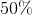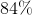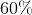Explanation:

Since we know that the population is in Hardy-Weinberg equilibrium and that there are only two alleles, we can use the Hardy-Weinberg equation to solve this problem: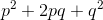Lets say thatrepresents the black allele, andrepresents the red allele. Since we know that red is recessive to black, only animals with two red alleles will be red. Fortunately, theportion of the equation is the only portion that deals with red animals (the other two variables are black: both homozygous dominant as well as heterozygous). This means thatis equal to the frequency of red animals in the population: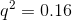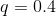Since we now know the frequency of the red allele in the population, we simply subtract it from one in order to find the frequency of the black allele, which turns out to be 0.6.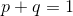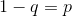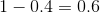### Example Question #1 : Other Evolution Principles

In a population that is in Hardy-Weinberg equilibrium, the frequency of homozygous dominant individuals is 0.36. What is the percentage of homozygous recessive individuals in the population?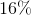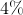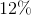Explanation:

The two equations pertaining to Hardy-Weinberg equilibrium are:In this second equation, each term refers to the frequency of a given genotype.is the homozygous dominant frequency,is the heterozygous frequency, andis the homozygous recessive frequency.

From the question, we know that: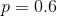We now know the dominant allele frequency. Using the other Hardy-Weinberg equation, we can find the recessive allele frequency: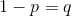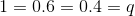Returning to our genotype frequency terms, we can use this recessive allele frequency to find the homozygous recessive frequency: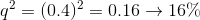### Example Question #1 : Evolutionary Factors

Which is not a necessary condition for the Hardy-Weinberg equation to be true?

Small population

No natural selection

Random mating

No mutations in the gene pool

No net migration of individuals into or out of the population

Small population

Explanation:

For the Hardy-Weinberg equation to be true, the population in question must be very large. This ensures that coincidental occurrences do not drastically alter allelic frequencies.

### Example Question #4 : Understanding Hardy Weinberg Equilibrium

A population is in Hardy-Weinberg equilibrium. The gene of interest has two alleles, with 16% of the population portraying the features of the recessive phenotype. What percentage of the population is heterozygous?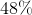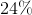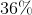Explanation:

Using the Hardy-Weinberg equilibrium equations, you can determine the answer.The value ofgives us the frequency of the dominant allele, while the value ofgives us the frequency of the recessive allele. The second equation corresponds to genotypes.is the homozygous dominant frequency,is the heterozygous frequency, andis the homozygous recessive frequency.

16% of the population shows the recessive phenotype, and therefore must carry the homozygous recessive genotype. We can use this information to solve for the recessive allele frequency.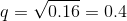We can use the value ofand the first Hardy-Weinberg equation to solve for.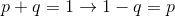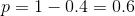Knowing bothand, you can use the second equation to find the percent of heterozygous organisms in the population.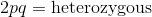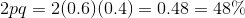### All GRE Subject Test: Biology Resources